# Find the curvature of a curve with a kissing circle using Processing

Taichi Kato

2021-06-03

To try to programatically solve this problem, I first set up a p5.js environment where I can plot a curve.

``````fr = 10
currentPoint=15
function setup() {
createCanvas(400, 400);
background(220);
frameRate(fr);
}
isDrawing = false;
points = [];
var e;
function draw() {
if(isDrawing){
points.push([mouseX, mouseY, points.length]);
ellipse(mouseX, mouseY, 2, 2);
if(points.length > 1){
line(mouseX, mouseY, points[points.length - 2], points[points.length - 2]);
}
}
if(points.length === currentPoint){
fill(255,123,0);
ellipse(points[currentPoint-2], points[currentPoint-2], 5, 5)
fill(255,0,0);
ellipse(points[currentPoint-1], points[currentPoint-1], 5, 5)
fill(255,123,0);
ellipse(points[currentPoint-3], points[currentPoint-3], 5, 5)
}
}
function mousePressed() {
if (isDrawing) {
isDrawing = false;
console.log(points)
} else {
isDrawing = true;
}
}
function keyPressed() {
if (keyCode === UP_ARROW) {
currentPoint += 1;
} else if (keyCode === DOWN_ARROW) {
currentPoint -= 1;
}

console.log('Current point: ', currentPoint);
}``````

Here, I have highlighted three points in red, which are the points I will use to create the kissing circle. Assuming that the three points do not lie on a straight line, we can find an unique circle which passes through them. We can use that circle, and its radius to approximate the curvature of the curve at the point.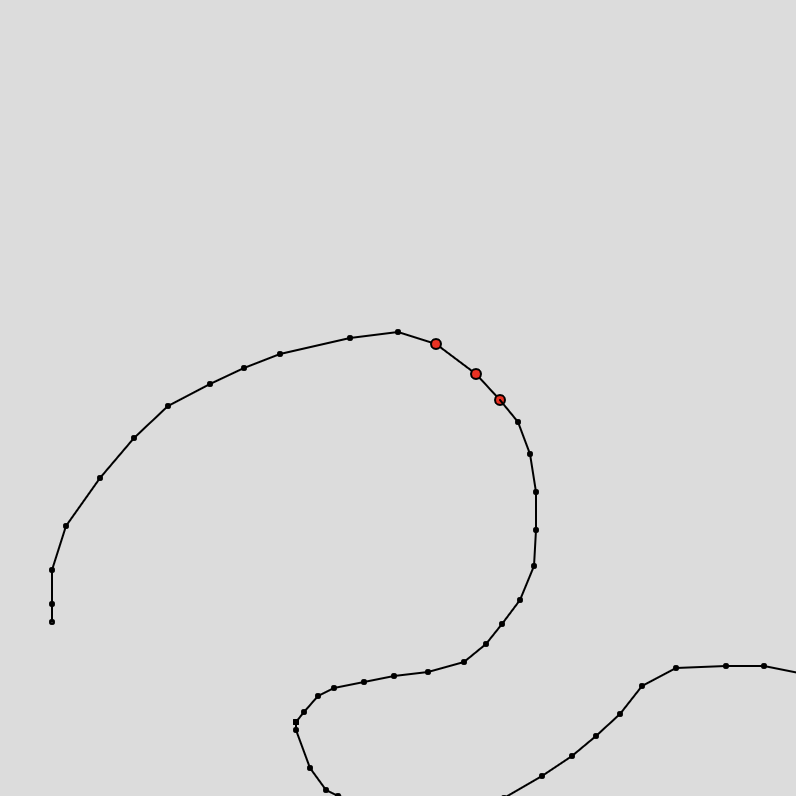I came across a stack exchange post which was basically tacking the same problem. However, to try to create a system which solves the system of equations which are provided here seemed rather difficult, given that they are non-linear. I tried to analytically solve the equation for r to create a function r(p1, p2, p3). However, I was not able to do this by hand.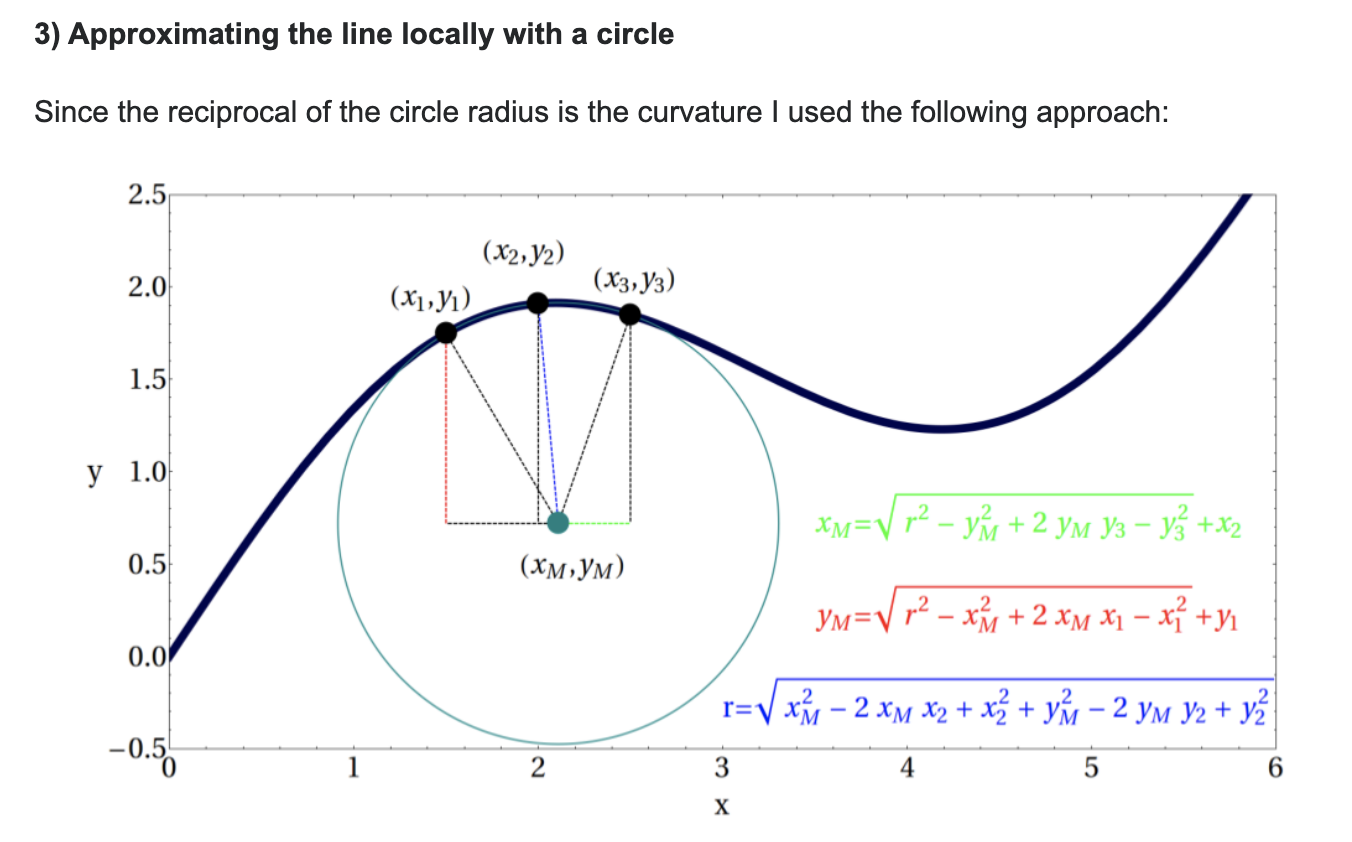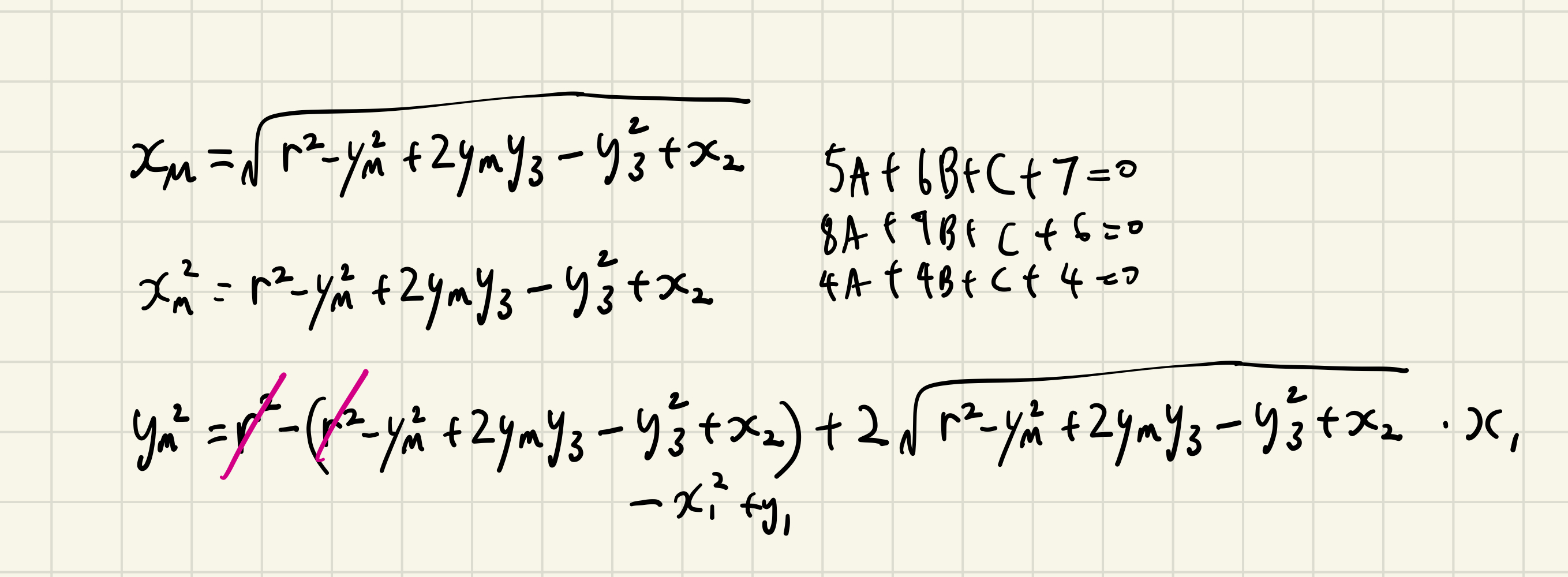(blah blah, I gave up on solving this.)

I then went back to thinking about ways in which I could find the radius, given the three points. I figured that I could create my own system of equations, such that it is easier to find the radius. Since all points lie on the circle, we could use the equation for a circle to create a set of equations which represents this circle.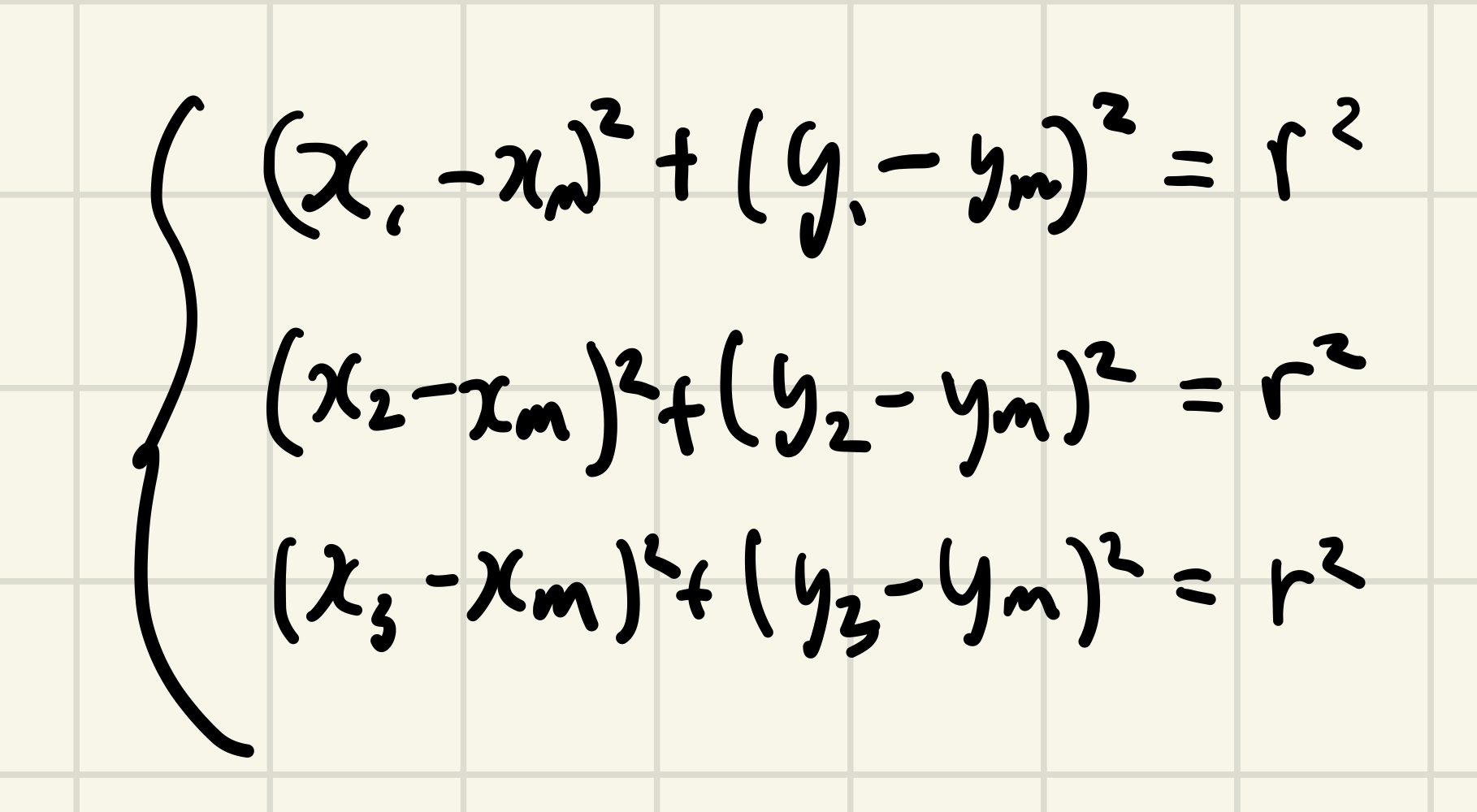This was definitely a step in the right direction, but this is still non-linear, so its difficult to solve. I could of course use numerical methods like Monte Carlo to approximate the values, but I figured it shouldn’t be too hard to find a way to turn this into a linear system of equations.

I was able to reduce it to a linear equation by creating three new variables A, B and C, which I can use to solve for `x_m`, `r` and `y_m` later on.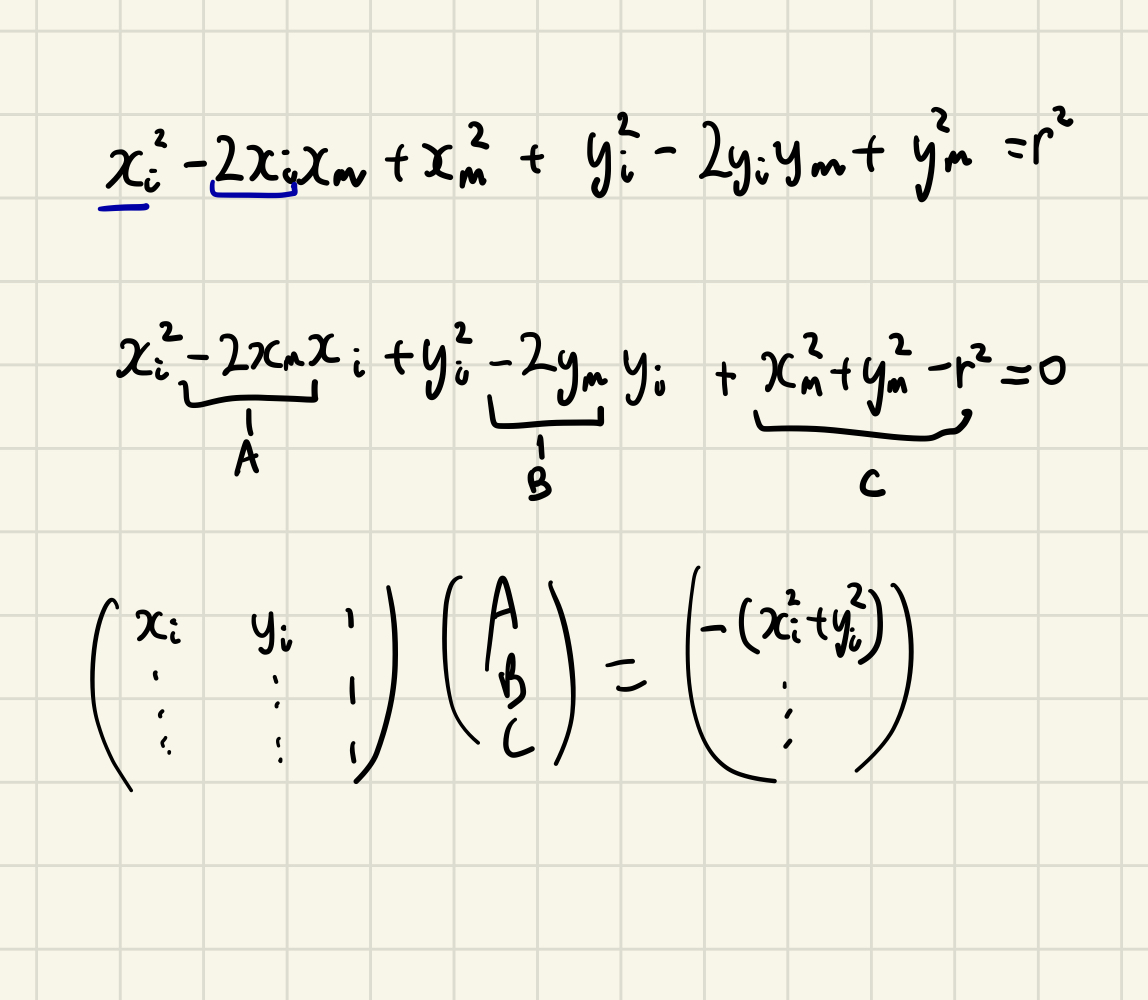Finally, I believe I can use these matrices to create a program to find a circle, and a curvature in the end.